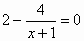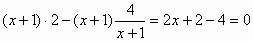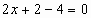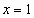# Translations of Encyclopedia about Mathematics

## Equations with Fractions

Introducing Fractions

Equations which contain at least one fraction containing a variable denominator are called equations with fractions. When solving equations with fractions, the same rules of calculation apply as with normal equations. Usually, when solving such equations, the first step is to find a common denominator. By multiplying equations with fractions having common denominators,

we manage to remove such denominators, as such giving us a normal equation which we can solve in the standard manner.

Example:

We are given the following equation with fractions:The first step is to multiply the equation by x + 1, to get:The second step is to solve the equation in the normal manner, to give us:| +2| :2Solving the equation in this manner gives us the solution: x = 1.

Translating Dutch Hungarian Translations Hungarian Dutch Translating Swedish Czech Translations Czech Swedish Translating RussianEnter your search terms Submit search form